Courses

# Test: Progression (AP And GP)- 4

## 15 Questions MCQ Test Quantitative Aptitude (Quant) | Test: Progression (AP And GP)- 4

Description
This mock test of Test: Progression (AP And GP)- 4 for Quant helps you for every Quant entrance exam. This contains 15 Multiple Choice Questions for Quant Test: Progression (AP And GP)- 4 (mcq) to study with solutions a complete question bank. The solved questions answers in this Test: Progression (AP And GP)- 4 quiz give you a good mix of easy questions and tough questions. Quant students definitely take this Test: Progression (AP And GP)- 4 exercise for a better result in the exam. You can find other Test: Progression (AP And GP)- 4 extra questions, long questions & short questions for Quant on EduRev as well by searching above.
QUESTION: 1

### How many terms are there in the AP 20, 25, 30,… 130.

Solution:

In order to count the number of terms in the AP, use the short cut:
[(last term – first term)/ common difference] + 1. In this case it would become:
[(130 – 20)/5] +1 = 23. Option (b) is correct.

QUESTION: 2

### Find the 1st term of an AP whose 8th and 12th terms are respectively 39 and 59.

Solution:

Since the 8th and the 12th terms of the AP are given as 39 and 59 respectively, the difference
between the two terms would equal 4 times the common difference. Thus we get 4d = 59 – 39 =
20. This gives us d = 5. Also, the 8th term in the AP is represented by a + 7d, we get:
a + 7d = 39 Æ a + 7 × 5 = 39 Æ a = 4. Option (c) is correct.

QUESTION: 3

### There is an AP 1, 3, 5…. Which term of this AP is 55?

Solution:

The number of terms in a series are found by: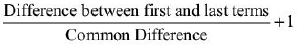QUESTION: 4

Find the lowest number in an AP such that the sum of all the terms is 105 and greatest term is 6 times the least.

Solution:

Trying Option (a),
We get least term 5 and largest term 30 (since the largest term is 6 times the least term).
The average of the A.P becomes (5 + 30)/2 = 17.5
Thus, 17.5 × n = 105 gives us:
to get a total of 105 we need n = 6 i.e. 6 terms in this A.P. That means the A.P. should look like:
5, _ , _ , _, _, 30.
It can be easily seen that the common difference should be 5. The A.P, 5, 10, 15, 20, 25, 30 fits the
situation.
The same process used for option (b) gives us the A.P. 10, 35, 60. (10 + 35 + 60 = 105) and in the
third option 15, 90 (15 + 90 = 105).
Hence, all the three options are correct.

QUESTION: 5

A sum of money kept in a bank amounts to ` 1240 in 4 years and ` 1600 in 10 years at simpleInterest. Find the sum.

Solution:

The difference between the amounts at the end of 4 years and 10 years will be the simple interest
on the initial capital for 6 years.
Hence, 360/6 = 60 =(simple interest.)
Also, the Simple Interest for 4 years when added to the sum gives 1240 as the amount.
Hence, the original sum must be 1000.

QUESTION: 6

The sum of the first 16 terms of an AP whose first term and third term are 5 and 15 respectively is

Solution: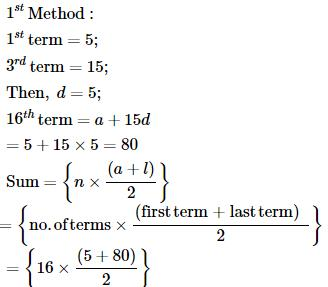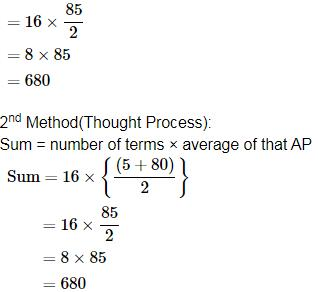QUESTION: 7

The least value of n for which the sum of the series 5 + 8 + 11… n terms is not less than 670 is

Solution:

Solve this question through trial and error by using values of n from the options:
For 19 terms, the series would be 5 + 8 + 11 + …. + 59 which would give us a sum for the series
as 19 × 32 = 608. The next term (20th term of the series) would be 62. Thus, 608 + 62 = 670
would be the sum to 20 terms. It can thus be concluded that for 20 terms the value of the sum of the
series is not less than 670. Option (a) is correct.

QUESTION: 8

How many terms are there in the GP 5, 20, 80, 320,… 20480?

Solution:

The series would be 5, 20, 80, 320, 1280, 5120, 20480. Thus, there are a total of 7 terms in the
series. Option (c) is correct.

QUESTION: 9

If the fifth term of a GP is 81 and first term is 16, what will be the 4th term of the GP?

Solution:

16r4 = 81 Æ r4 = 81/16 Æ r = 3/2. Thus, 4th term = ar3 = 16 × (3/2)3 = 54. Option (c) is correct

QUESTION: 10

The sum of three numbers in a GP is 14 and the sum of their squares is 84. Find the largestnumber.

Solution:

Visualising the squares below 84, we can see that the only way to get the sum of 3 squares as 84
is: 22 + 42 + 82 = 4 + 16 + 64. The largest number is 8. Option (a) is correct.

QUESTION: 11

How many natural numbers between 300 to 500 are multiples of 7?

Solution:

The series will be 301, 308, …….. 497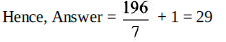QUESTION: 12

If a man saves ` 4 more each year than he did the year before and if he saves ` 20 in the first year,after how many years will his savings be more than ` 1000 altogether?

Solution:

We need the sum of the series 20 + 24 + 28 to cross 1000. Trying out the options, we can see that
in 20 years the sum of his savings would be: 20 + 24 + 28 + … + 96. The sum of this series would
be 20 × 58 =1160. If we remove the 20th year we will get the series for savings for 19 years. The
series would be 20 + 24 + 28 + …. 92. Sum of the series would be 1160 – 96 = 1064. If we
remove the 19th year’s savings the savings would be 1064 – 92 which would go below 1000.
Thus, after 19 years his savings would cross 1000. Option (a) is correct.

QUESTION: 13

The 4th and 10th term of an GP are 1/3 and 243 respectively. Find the 2nd term.

Solution: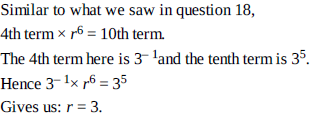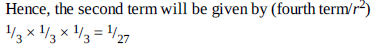[Note: To go forward in a G.P. you multiply by the common ratio, to go backward in a G.P. you
divide by the common ratio.]

QUESTION: 14

The sum of 5 numbers in AP is 30 and the sum of their squares is 220. Which of the following is the third term?

Solution:

Since the sum of 5 numbers in AP is 30, their average would be 6. The average of 5 terms in AP is
also equal to the value of the 3rd term (logic of the middle term of an AP). Hence, the third term’s
value would be 6. Option (b) is correct.

QUESTION: 15

The sum of the first four terms of an AP is 28 and sum of the first eight terms of the same AP is 88.Find the sum of the first 16 terms of the AP?

Solution:

Think like this:
The average of the first 4 terms is 7, while the average of the first 8 terms must be 11.
Now visualize this :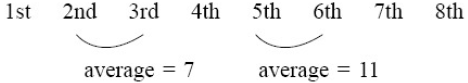Hence, d = 4/2 = 2 [Note: understand this as a property of an A.P.]
Hence, the average of the 6th and 7th terms = 15 and the average of the 8th and 9th term = 19
But this (19) also represents the average of the 16 term A.P.
Hence, required answer = 16 × 19 = 304.